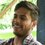# Prove This beautiful result !

$\alpha \quad ,\quad \beta \quad >\quad 0\quad \quad \& \quad \quad \theta \quad \in \quad R\\ \\ f\left( \theta \right) \quad =\quad \quad \alpha \sec ^{ 2 }{ \theta } \quad +\quad \beta \csc ^{ 2 }{ \theta } \quad +\quad \sqrt { { \alpha }^{ 2 }\sec ^{ 4 }{ \theta } \quad +\quad { \beta }^{ 2 }\csc ^{ 4 }{ \theta } }$.

Then Prove That Minimum Value of $f\left( \theta \right)$ is :

${ f\left( \theta \right) }_{ min }\quad =\quad 2(\alpha \quad +\quad \beta \quad +\quad \sqrt { 2\alpha \beta } )$.

Extra credit: Just observe it !
( It's Look ugly But Believe me in actual it is very very Beautiful question )

Following tool of mathematics are may Helpful Here Like : Substitution , Geometrical interpretation , AM - GM , Circles , Straight lines , Polar substitution etc, !!Note by Deepanshu Gupta
6 years, 7 months ago

This discussion board is a place to discuss our Daily Challenges and the math and science related to those challenges. Explanations are more than just a solution — they should explain the steps and thinking strategies that you used to obtain the solution. Comments should further the discussion of math and science.

When posting on Brilliant:

• Use the emojis to react to an explanation, whether you're congratulating a job well done , or just really confused .
• Ask specific questions about the challenge or the steps in somebody's explanation. Well-posed questions can add a lot to the discussion, but posting "I don't understand!" doesn't help anyone.
• Try to contribute something new to the discussion, whether it is an extension, generalization or other idea related to the challenge.

MarkdownAppears as
*italics* or _italics_ italics
**bold** or __bold__ bold
- bulleted- list
• bulleted
• list
1. numbered2. list
1. numbered
2. list
Note: you must add a full line of space before and after lists for them to show up correctly
paragraph 1paragraph 2

paragraph 1

paragraph 2

[example link](https://brilliant.org)example link
> This is a quote
This is a quote
    # I indented these lines
# 4 spaces, and now they show
# up as a code block.

print "hello world"
# I indented these lines
# 4 spaces, and now they show
# up as a code block.

print "hello world"
MathAppears as
Remember to wrap math in $$ ... $$ or $ ... $ to ensure proper formatting.
2 \times 3 $2 \times 3$
2^{34} $2^{34}$
a_{i-1} $a_{i-1}$
\frac{2}{3} $\frac{2}{3}$
\sqrt{2} $\sqrt{2}$
\sum_{i=1}^3 $\sum_{i=1}^3$
\sin \theta $\sin \theta$
\boxed{123} $\boxed{123}$

Sort by:

Okay Now its time to give Hint :

use Substitution : $a\quad =\quad \alpha \sec ^{ 2 }{ \theta } \\ \\ b\quad =\quad \beta \csc ^{ 2 }{ \theta }$.

- 6 years, 7 months ago

Ohh ! Now I got something

is it mean :

$f(\theta )\quad =\quad a\quad +\quad b\quad +\quad \sqrt { { a }^{ 2 }\quad +\quad { b }^{ 2 } }$.

I think it is Perimeter of right angle triangle ?

Am i going Correct ?

- 6 years, 7 months ago

Yes ! You are on right Path ! Keep Trying You will find the way :)

- 6 years, 7 months ago

good Question,,!! rememberedme to high school..!! :D

- 6 years, 7 months ago

See Unravelling an inequality problem by Calvin Lin.

- 6 years, 7 months ago

That's certainly one way to motivate the solution.

Recognizing and relating patterns is helpful in approaching such problems.

Staff - 6 years, 7 months ago

@Calvin Lin Sir I solved in this way :(Similar to your Awesome Technique )imgur

Let $P\quad =\quad a\quad +\quad b\quad +\quad \sqrt { { a }^{ 2 }\quad +\quad { b }^{ 2 } }$.

also Let $\sqrt { { a }^{ 2 }\quad +\quad { b }^{ 2 } } \quad =\quad { C }_{ 1 }\quad +\quad { C }_{ 2 }$.

$P\quad =\quad (\quad a\quad +\quad { C }_{ 2 }\quad )\quad +\quad (\quad b\quad +\quad { C }_{ 1 }\quad )\quad$.

Now For Minimum perimeter P we use AM-GM inequality

$P\quad \ge \quad 2\sqrt { (\quad a\quad +\quad { C }_{ 2 }\quad )(\quad b\quad +\quad { C }_{ 1 }\quad ) } \\ \\ { P }_{ min }\quad =\quad 2\sqrt { (\quad a\quad +\quad { C }_{ 2 }\quad )(\quad b\quad +\quad { C }_{ 1 }\quad ) } \quad \\$

If and only iff :

1)- GM must be constant

2)- Equality of variable must be attained

$\\ \bullet \quad (\quad a\quad +\quad { C }_{ 2 }\quad )(\quad b\quad +\quad { C }_{ 1 }\quad )\quad =\quad constant\\ \\ \bullet \quad (\quad a\quad +\quad { C }_{ 2 }\quad )\quad =\quad (\quad b\quad +\quad { C }_{ 1 }\quad )\quad =\quad r\quad (say)\\ \\ { P }_{ min }\quad =\quad 2r\quad$.

Now Same as Yours !

Is This is correct ? Calvin Sir ?

- 6 years, 7 months ago

what about area at this stage?@Deepanshu Gupta

- 6 years, 7 months ago

I don't think that we can do more for calculating area from above information in this particular question . what do you think ? @sandeep Rathod

- 6 years, 7 months ago

ohh is it a famous problem? I don't know about this . Actually this question was giving to me by a friend of me as a challange !

So I Liked it after solving it So i share this ! ,

But i solved it in slightly different manner as that calvin sir posted ( also my friend also help me little in how to starting this problem )

- 6 years, 7 months ago

So what is your Slightly different approach Can you post it ! please Thanks ! @Deepanshu Gupta

- 6 years, 7 months ago

I had Posted it @KARAN SHEKHAWAT

But I'am not 100% sure that it is correct or not !

- 6 years, 7 months ago